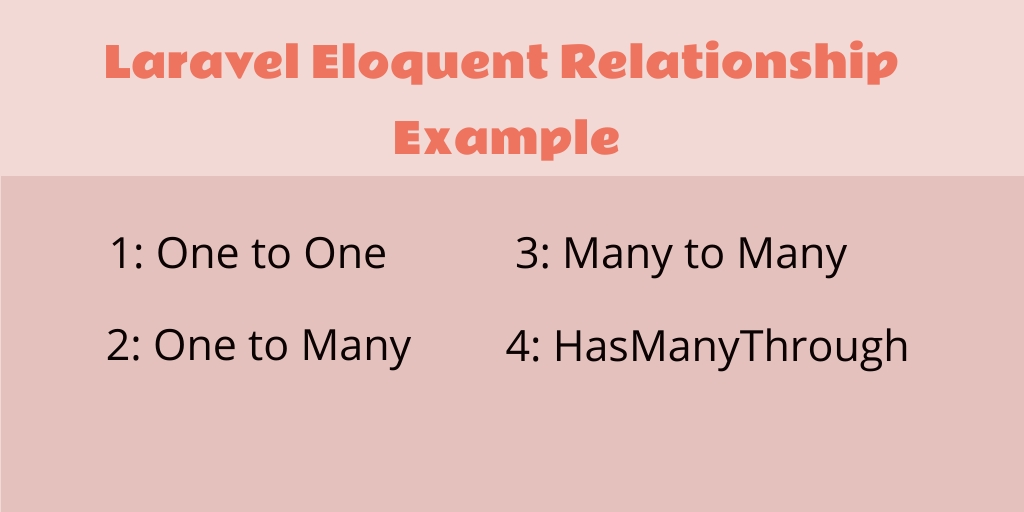Laravel 22-Dec-2020

### Laravel Eloquent Relationships Example

Laravel Eloquent Relationships example. This tutorial represents to you, how to create and use eloquent relationships in laravel apps with example.

## Laravel Eloquent Relationships

There are following types of eloquent relationships in laravel:

Learn one by one eloquent relationship with example in laravel:

### Laravel eloquent one to one example

One to one relationship is one of basic relationships. For example, a Post model would be associated with a content model. To illustrate this relationship, we can create a post_content() method within the Post model and call the hasOne() method to relate the Content model.

<?php

namespace App;

use Illuminate\Database\Eloquent\Model;

class Post extends Model
{
public function post_content()
{
return \$this->hasOne('App\Content');
}
}

It is important to note that Eloquent establishes a foreign key based on the model name and should have a matching value id. Here post_id is the foreign_key of Content, So in order to create the relation.

#### The inverse of One to One Relationship Example

So far, we can access the content from the post. Let us now create an inverse relationship on the content model so that the post can access the model from it. To do this, we can use the belongsTo method for getting the post data on the content model.

<?php

namespace App;

use Illuminate\Database\Eloquent\Model;

class Content extends Model
{
public function post()
{
return \$this->belongsTo('App\Post');
}
}

We can now access the post content using the relation method like this:

`\$content = Post::find(10)->post_content;`

### Laravel eloquent one to many example

One of many relationships determines a relationship where one single model owns the number of the other model. For example, a blog author may have many post.

A single author can have written many post articles. Let’s take an example of one to many relationships.

<?php

namespace App;

use Illuminate\Database\Eloquent\Model;

class Author extends Model
{
...
public function post()
{
return \$this->hasMany('App\Post');
}
}

We can then access the collection of post article by using the post() method as.

Using this, we get all the posts of a single author. Here we need to get the author details of a single post,for each post there a single author, within the Post model includes belongsTo relation.

```\$posts = App\Author::find(10)->post()->get();

foreach (\$posts as \$post) {
//do something
}```

#### Inverse One to Many Relationship Example

Since we can now access all the post articles of an author, it is time to allow a post article to access its parent model (Author model) or access author details by using the post model. The inverse relationship of both One to One and One to Many works the same way. Both use the belongsTo method to define the inverse relation. Thus we can define one as:

<?php

namespace App;

use Illuminate\Database\Eloquent\Model;

class Post extends Model
{
public function author()
{
return \$this->belongsTo('App\Author');
}
}

Using this, Here we need to get the author details by using posts,for each post there a single author, within the Post model includes belongsTo relation.

`\$post->author->author_name;`

### Laravel Eloquent Many to Many Relationship Example

Each post have many tag and each tag can have many post.

To define many to many relationships, we use belongsToMany() method.

<?php

namespace App;

use Illuminate\Database\Eloquent\Model;

class Post extends Model
{
...
public function tags()
{
return \$this->belongsToMany('App\Tag');
}
}

We can now access the tags in post model:

```\$post = App\Post::find(8);

foreach (\$post->tags as \$tag) {
//do something
}```

#### The inverse of Many to Many Relationship Example

The inverse of a many to many relationships can be defined by simply calling the similar belongsToMany method on the reverse model. We can illustrate that by defining posts() method in Tag model as:

<?php

namespace App;

use Illuminate\Database\Eloquent\Model;

class Tag extends Model
{
public function posts()
{
return \$this->belongsToMany('App\Post');
}
}

We can now access the post in tag model:

```\$tag = App\Tag::find(8);

foreach (\$tag->posts as \$post) {
//do something
}```

### Laravel Eloquent HasMany Through Relationship Example

The “has-many-through” relationship provides a convenient shortcut for accessing distant relations via an intermediate relation. For example, a `Country` model might have many `Post` models through an intermediate `User` model. In this example, you could easily gather all blog posts for a given country. Let’s look at the tables required to define this relationship:

<?php

namespace App;

use Illuminate\Database\Eloquent\Model;

class Country extends Model
{
/**
* Get all of the posts for the country.
*/
public function posts()
{
return \$this->hasManyThrough('App\Post', 'App\User');
}
}

## Conclusion

In this tutorial, you have learned how to use one to one, one to many, many to many and hasManyThrough relationships with example.